Stan Development Team

The bayesplot package provides a variety of ggplot2-based plotting functions for use after fitting Bayesian models (typically, though not exclusively, via Markov chain Monte Carlo). The package is designed not only to provide convenient functionality for users, but also a common set of functions that can be easily used by developers working on a variety of packages for Bayesian modeling, particularly (but not necessarily) packages powered by RStan (the R interface to Stan). Examples of packages that will soon (or already are) using bayesplot are rstan itself, as well as the rstan-dependent rstanarm and brms packages for applied regression modeling.

## Plotting functionality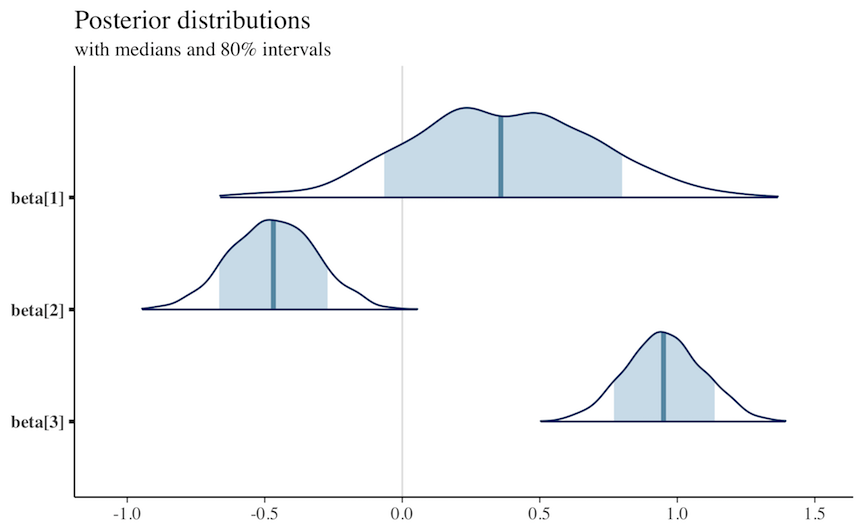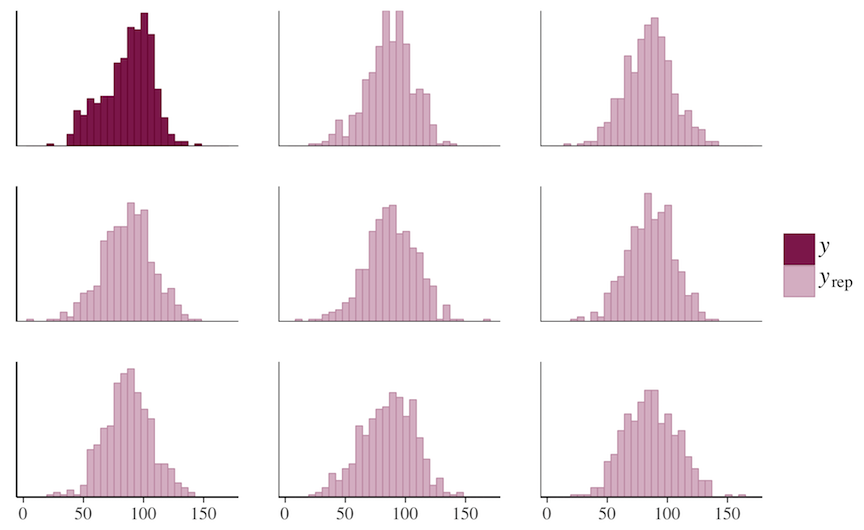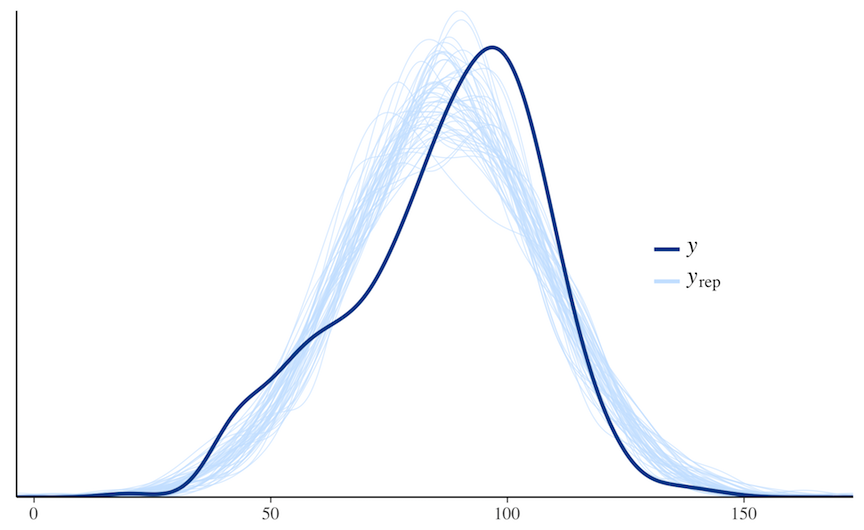The plotting functions in bayesplot are organized into several modules:

• MCMC: Visualizations of Markov chain Monte Carlo (MCMC) simulations generated by any MCMC algorithm as well as diagnostics. There are also additional functions specifically for use with models fit using the No-U-Turn Sampler (NUTS).

• PPC: Graphical (posterior or prior) predictive checks (PPCs).

• PPD: Plots of (posterior or prior) predictive distributions without comparisons to observed data.

## Resources

theme_default() for the default ggplot theme used by bayesplot and bayesplot_theme_set() to change it.

bayesplot-colors to set or view the color scheme used for plotting.

ggplot2::ggsave() for saving plots.

## Examples

# A few quick examples (all of the functions have many examples
# on their individual help pages)

# MCMC plots
x <- example_mcmc_draws(params = 5)
mcmc_intervals(x, prob = 0.5)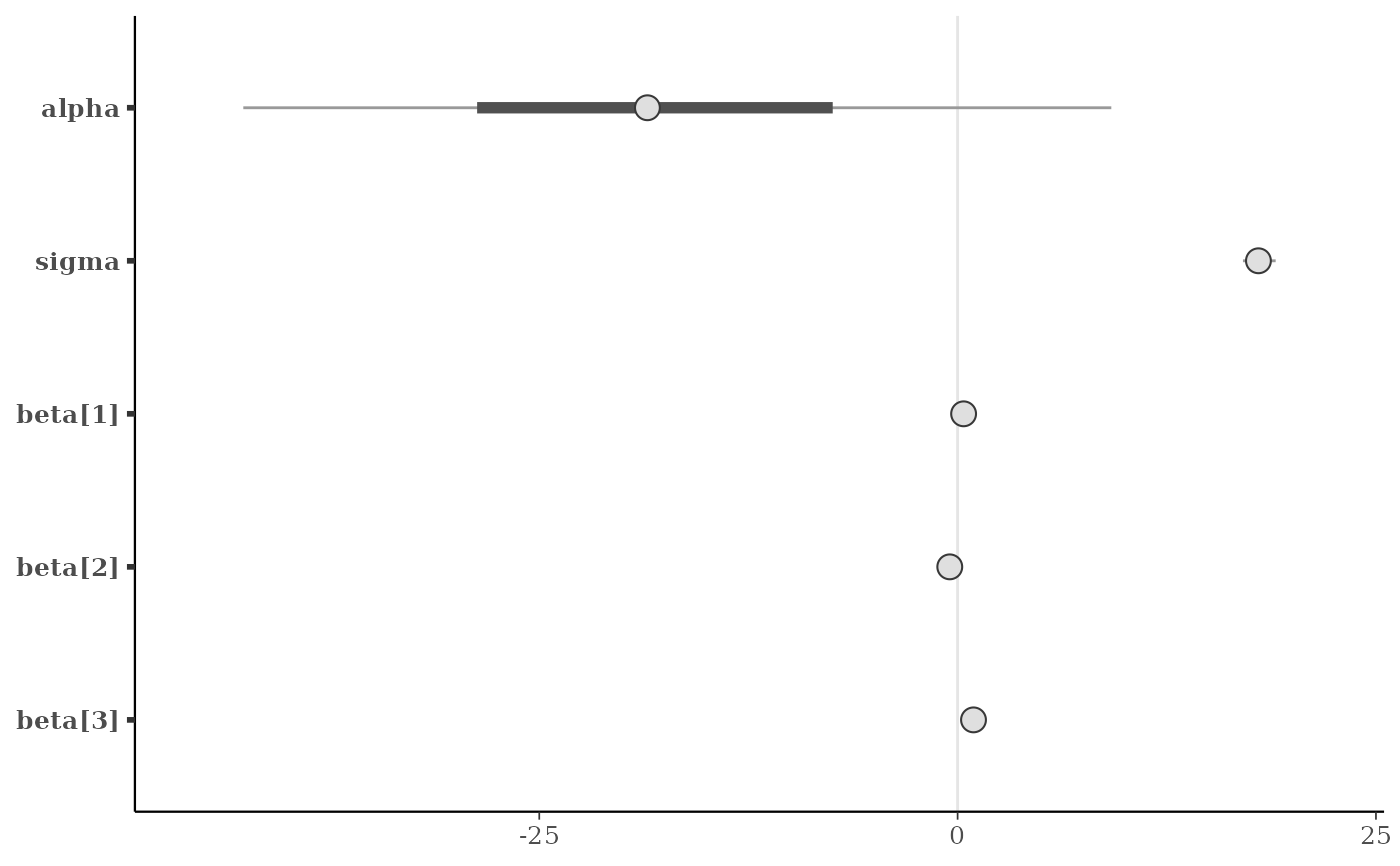mcmc_intervals(x, regex_pars = "beta")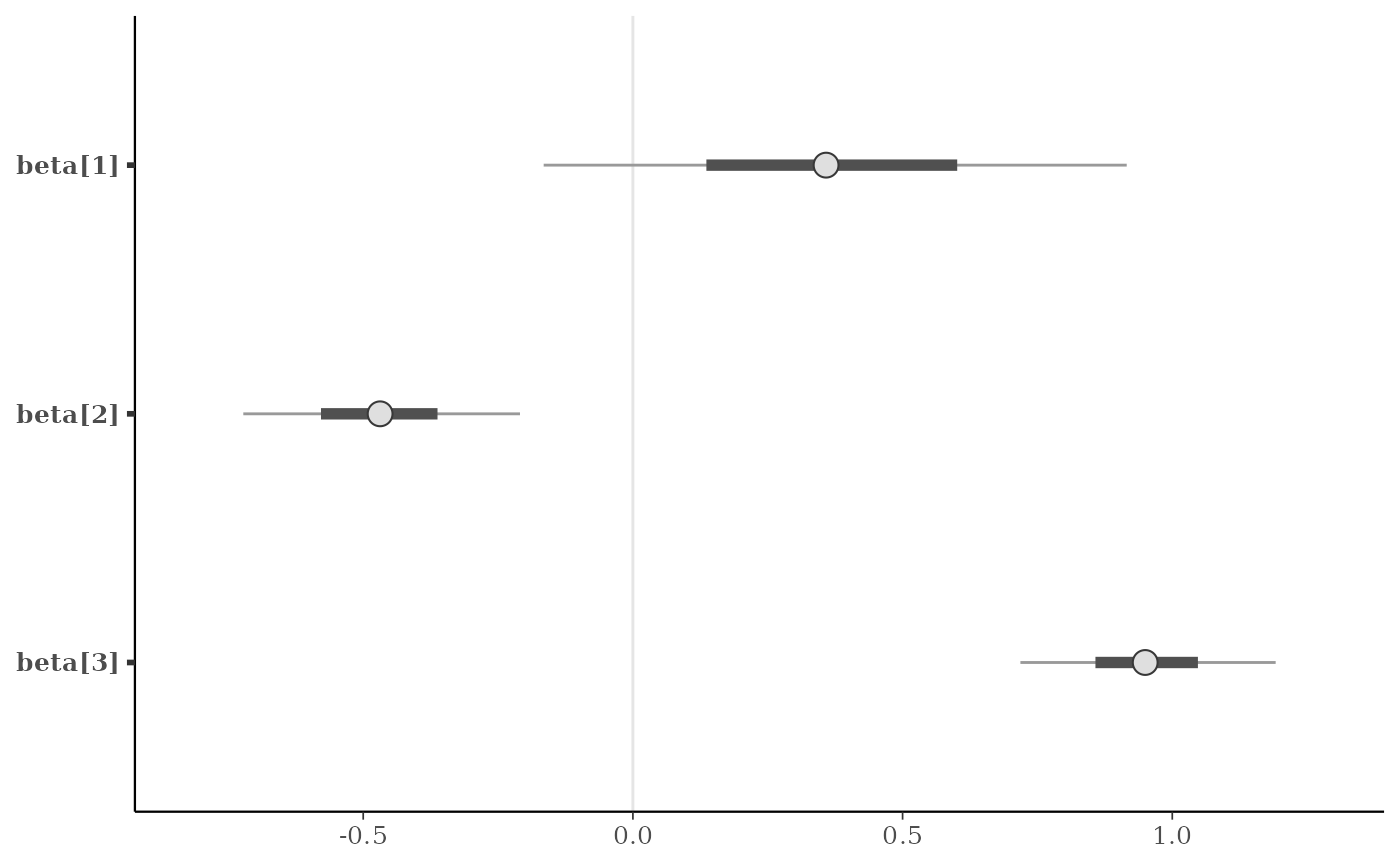color_scheme_set("purple")
mcmc_areas(x, regex_pars = "beta", prob = 0.8)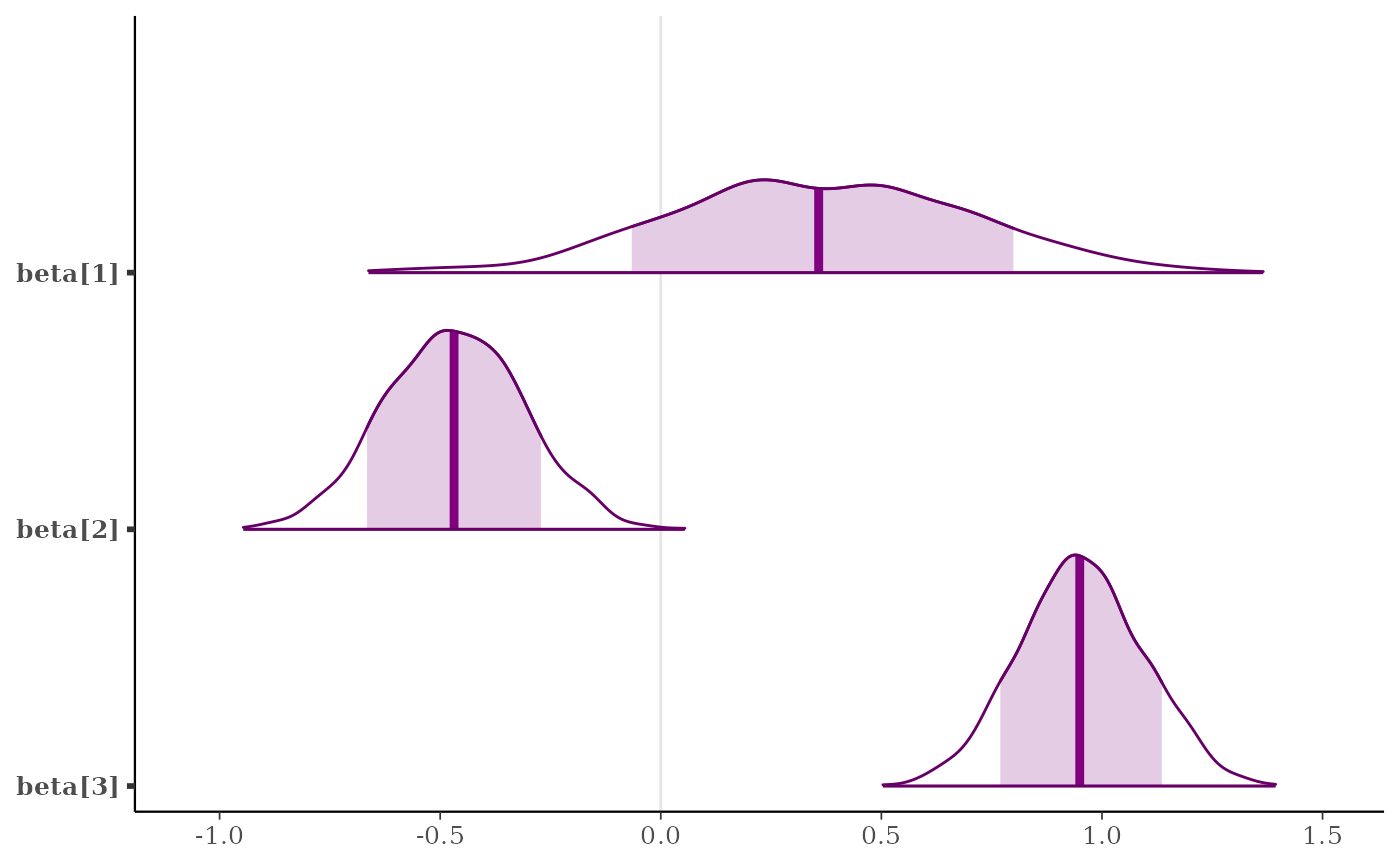color_scheme_set("mix-blue-red")
mcmc_trace(x, pars = c("alpha", "sigma"),
facet_args = list(nrow = 2))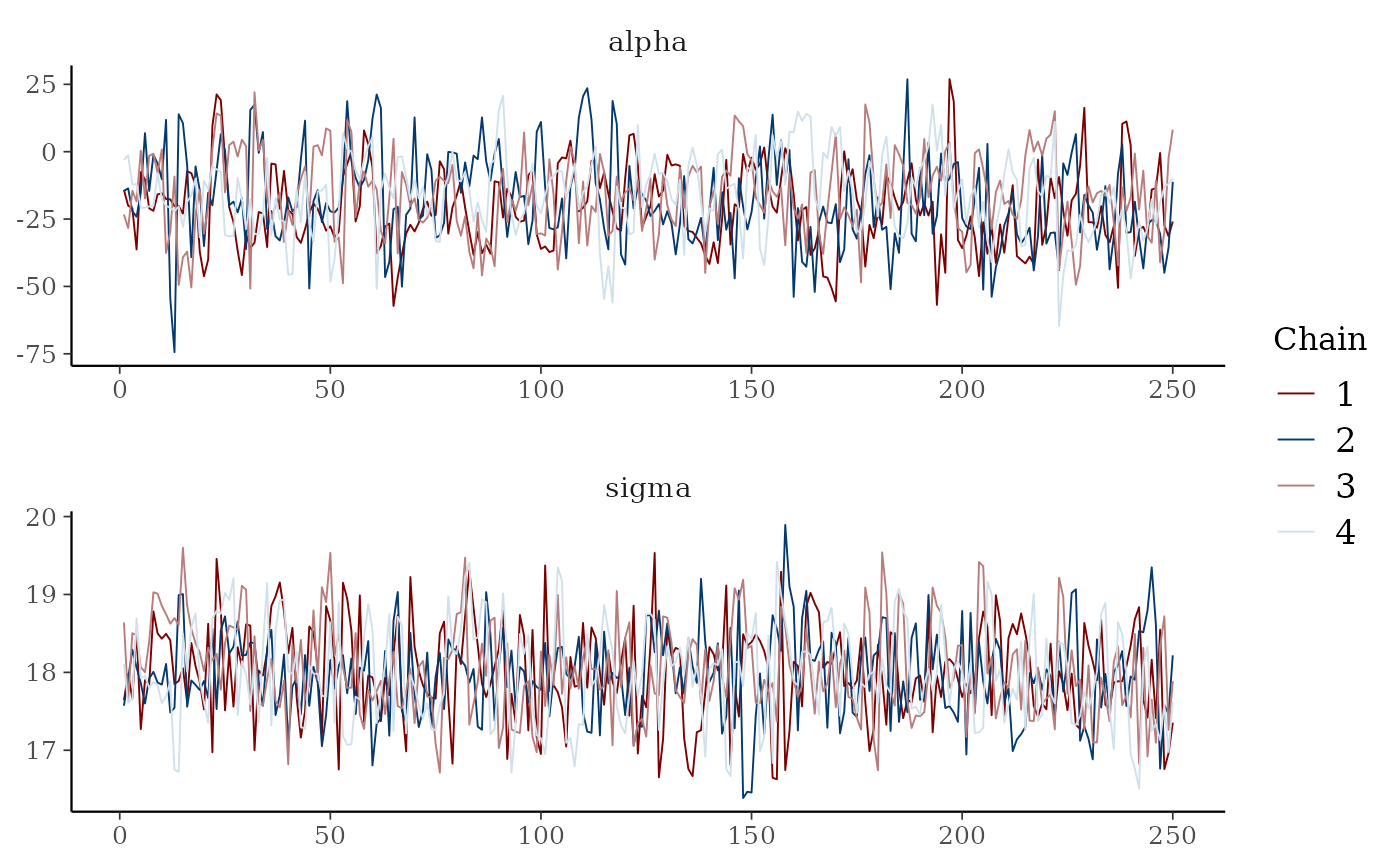color_scheme_set("brightblue")
mcmc_scatter(x, pars = c("beta", "sigma"),
transformations = list(sigma = "log"))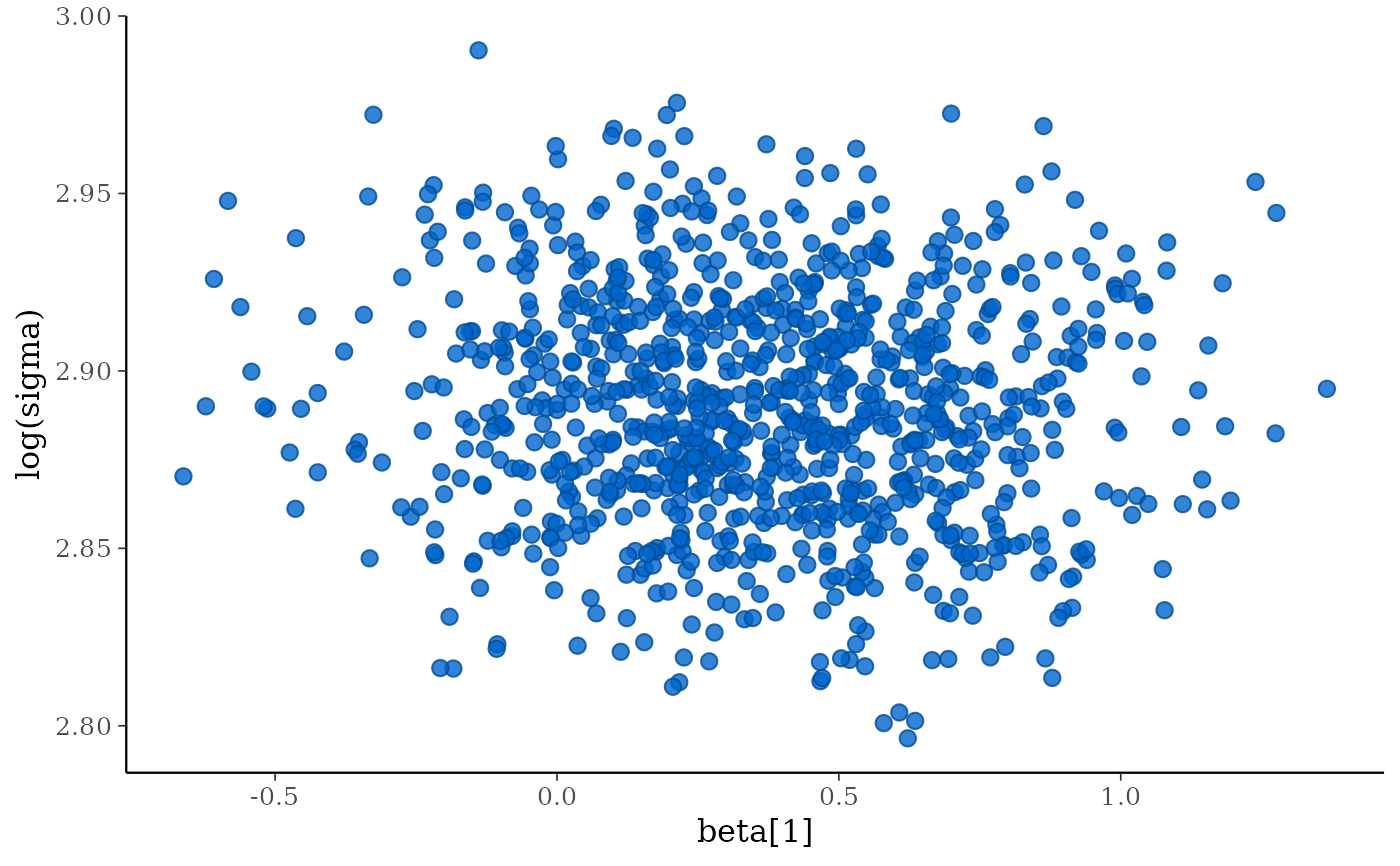# Graphical PPCs
y <- example_y_data()
yrep <- example_yrep_draws()
ppc_dens_overlay(y, yrep[1:50, ])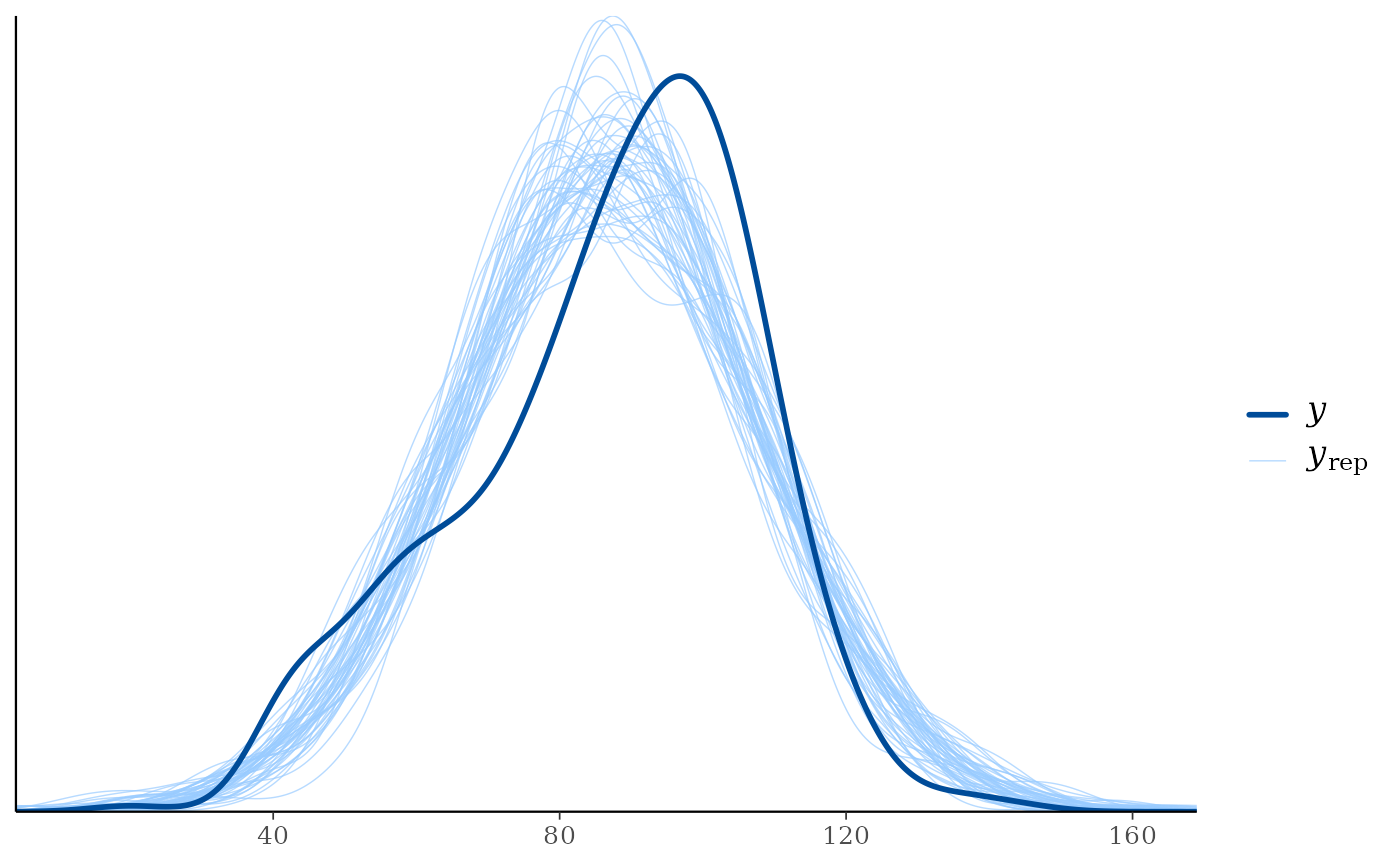# \donttest{
color_scheme_set("pink")
ppc_stat(y, yrep, stat = "median") + grid_lines()
#> stat_bin() using bins = 30. Pick better value with binwidth.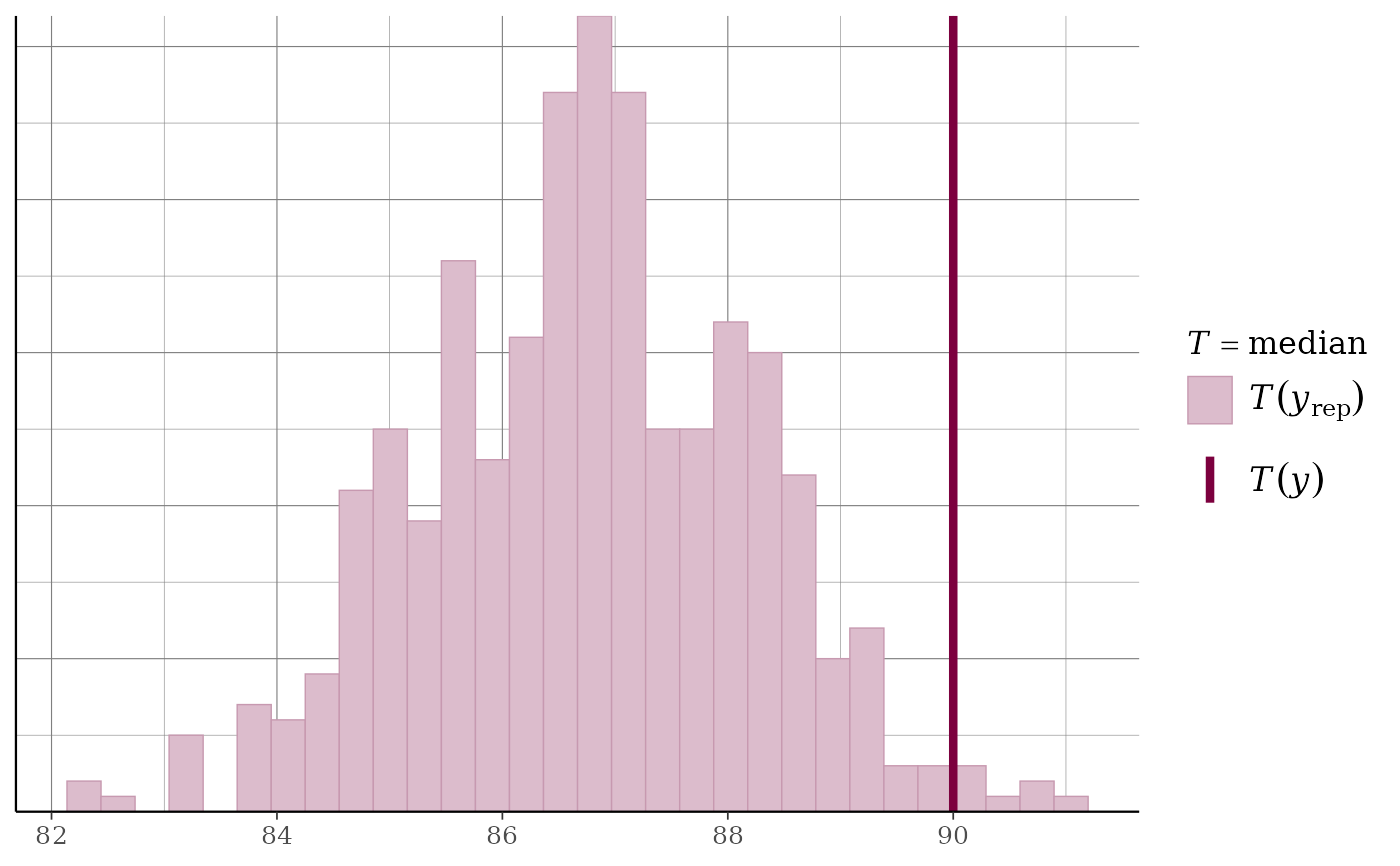ppc_hist(y, yrep[1:8, ])
#> stat_bin() using bins = 30. Pick better value with binwidth.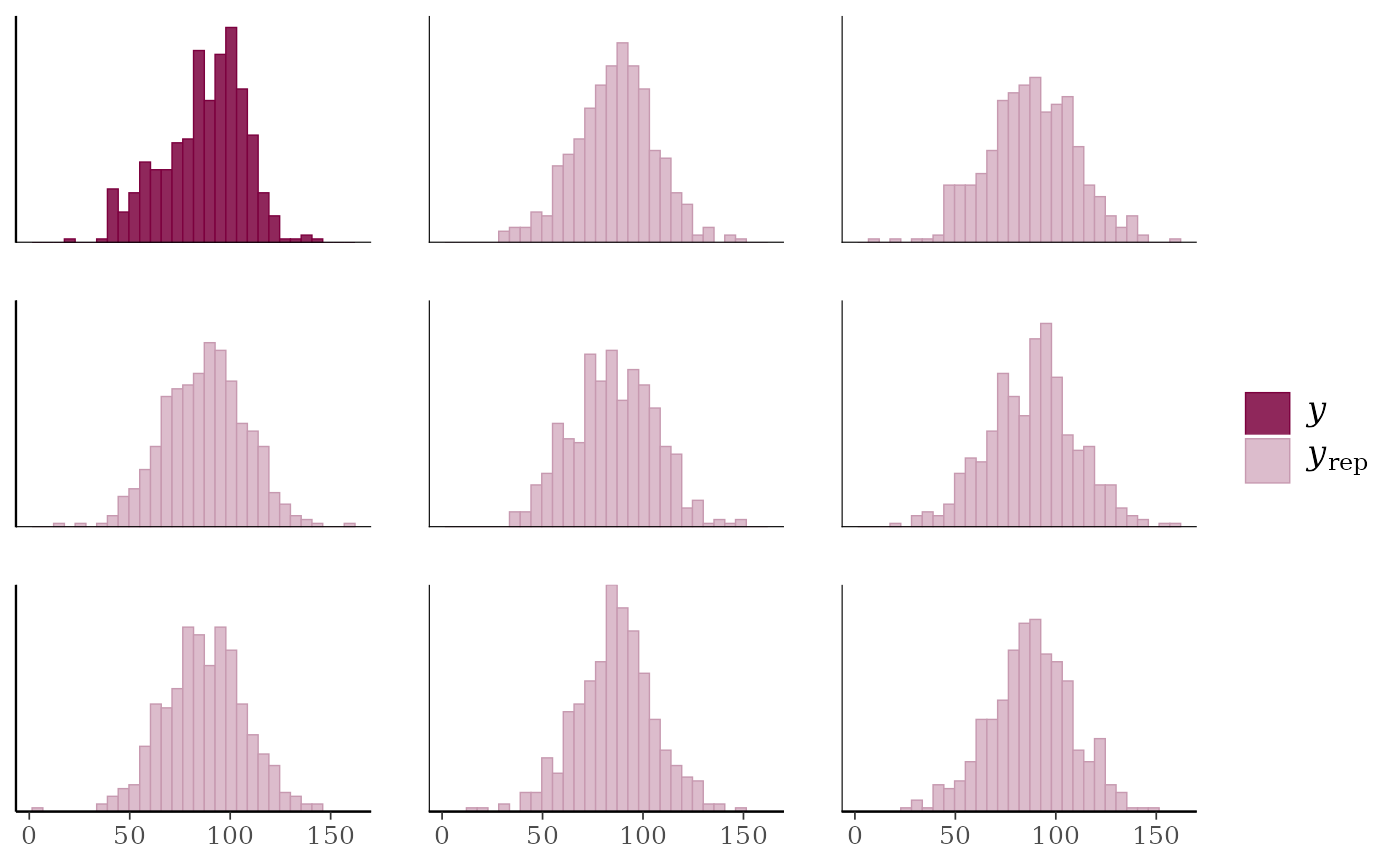# Same plots but without y (using ppd_ instead of ppc_)
bayesplot_theme_set(ggplot2::theme_gray())
ypred <- yrep
ppd_dens_overlay(ypred[1:50, ])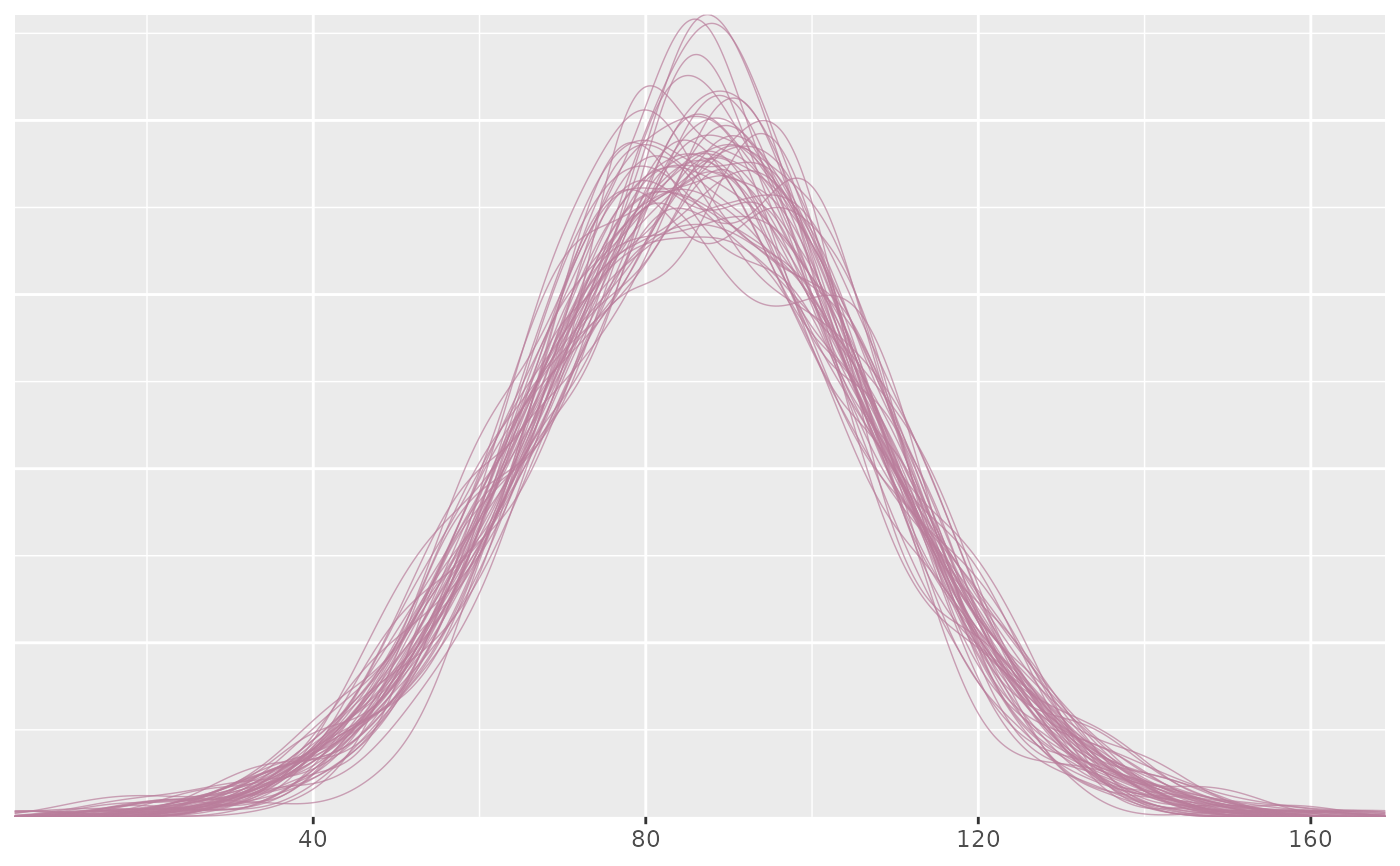ppd_stat(ypred, stat = "median") + grid_lines()
#> stat_bin() using bins = 30. Pick better value with binwidth.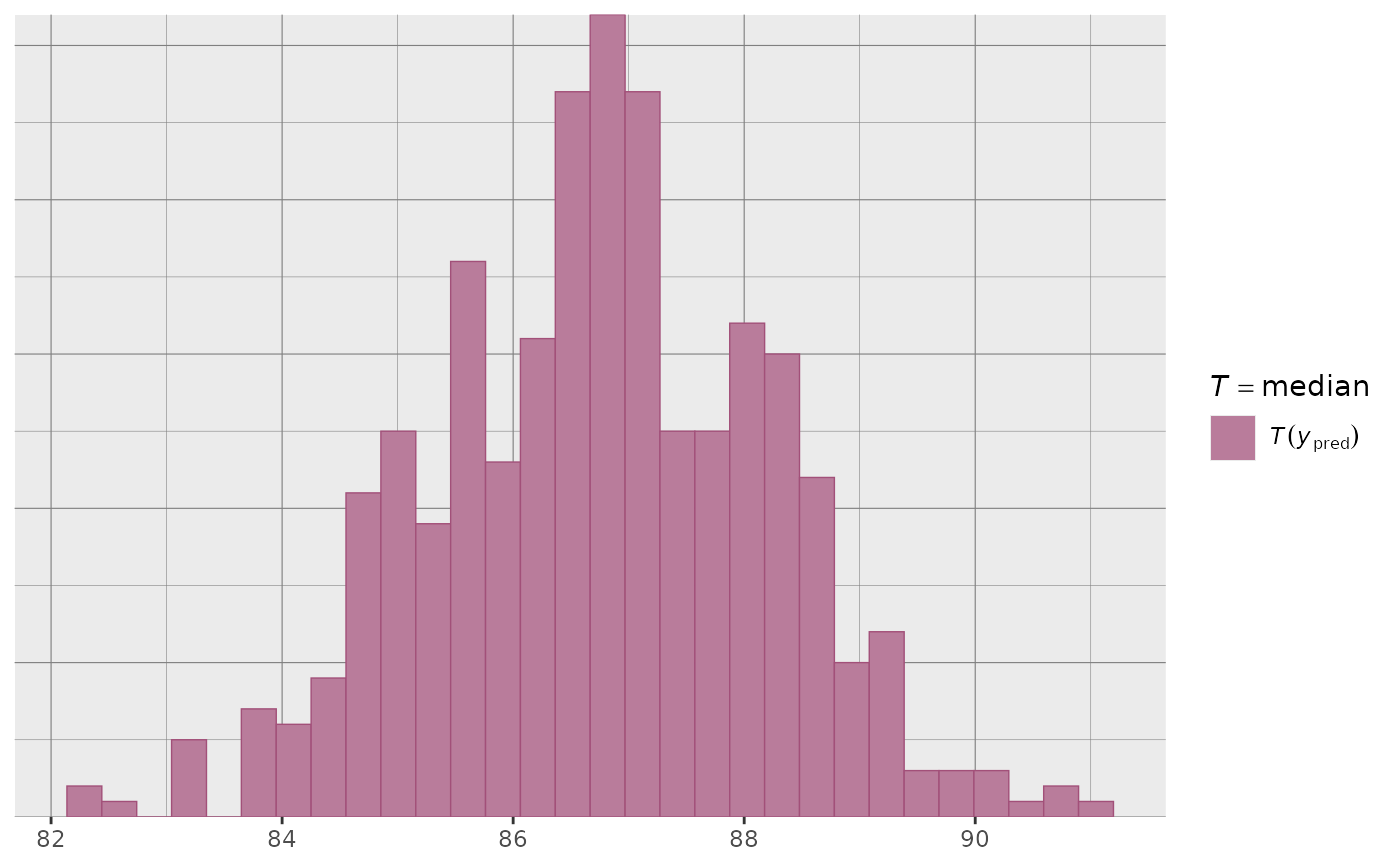ppd_hist(ypred[1:8, ])
#> stat_bin() using bins = 30. Pick better value with binwidth.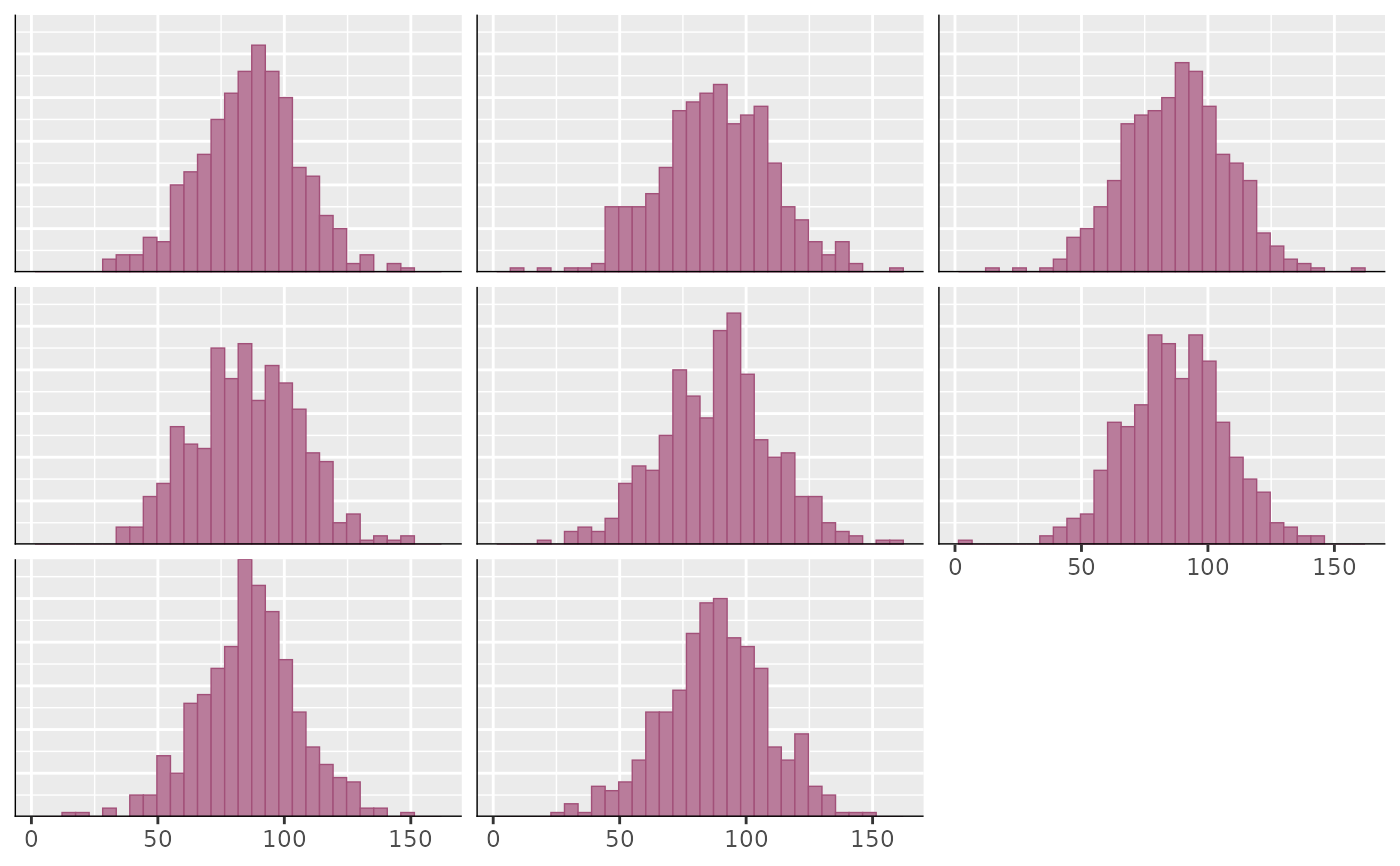# }